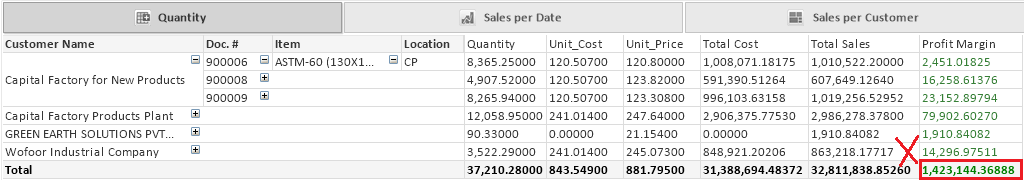# New to QlikView

Discussion board where members can get started with QlikView.

Announcements
Modernize Your QlikView Deployment webinar, Nov. 3rd. REGISTER
cancel
Showing results for
Did you mean:
HighlightedCreator II

## Calculation in pivot table

why the calculation in pivot table is not accurate it give a wrong number, but in straight table it's right !!1 Solution

Accepted Solutions
HighlightedSpecialist II

hi there,

It depends on what is the level of your aggrigation,

use the following exp

=sum(aggr(

((sum(QTY) + (vQty2)) * (sum([Unit Price])* (1+vSales2/100)))

-

(

((sum([Unit Cost]) * sum(QTY))) * (1+vCost2/100)

)

,

[Customer Name],

[SOP Number],

Item_Description,

[S_Location Code]

))

6 Replies
HighlightedMVP

Provide sample application or sample data to work...

HighlightedCreator II

qlik example

HighlightedCreator II

I put it in last comment.

regards.

HighlightedMVP

There are so many information and objects in your qvw. We need to have only those two where you are trying to match!

HighlightedSpecialist II

hi there,

It depends on what is the level of your aggrigation,

use the following exp

=sum(aggr(

((sum(QTY) + (vQty2)) * (sum([Unit Price])* (1+vSales2/100)))

-

(

((sum([Unit Cost]) * sum(QTY))) * (1+vCost2/100)

)

,

[Customer Name],

[SOP Number],

Item_Description,

[S_Location Code]

))

HighlightedCreator II

Thanks a lot.